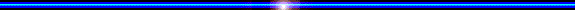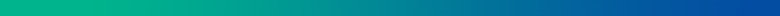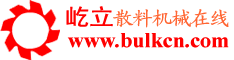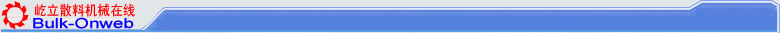您的位置：首页->技术论文->正文

 Measurement and Analysis upon the Gravity center Position of the Luffing system of stacker and reclaimer in whole luffing style the People's Republic of China LiYimin    Key words: stacker and reclaimer; gravity center of luffing system measurement Stacker and bucket wheel reclaimer could be in whole or partial luffing style when applying hydraulic cylinders to drive the luffing system. The whole luffing style is the slewing platform set with two pivots to support the whole luffing system, in addition one or two cylinders connecting the slewing platform and the hoisting system to adjust the luffing angle. In the course of luffing , the rotating angles around the axis of the two pivots on the slewing platform for any parts of the general luffing system, such as the bucket wheel, boom and counter-weight etc. are equal, meaning that the upper part is a whole part rotating around the two pivots. For common styles of whole luffing system see fig 1. and fig 2.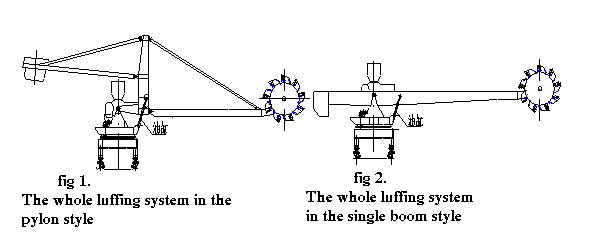In the course of using and maintenance, the users need to know and master the position as well as the changing law of gravity center of the whole machine or the luffing system so as to keep routine maintenance, due to various kinds of reasons. The position as well as the changing law of gravity center of the general luffing system could be known by means of measuring then calculation. 1. The measurement upon unbalanced torque The unbalanced torque which is the product of the distance between the gravity center of the hoisting system and the axis of the two pivots on the slewing platform and the total weight of the hoisting system, could be got by means of measuring the balanced-to-ground force at the bucket wheel or the pressure of the cylinder and then calculation. 1.1 Measuring the unbalanced torque by means of testing the balanced-to-ground force at the bucket wheel. Applying instruments such as automobile crane, electronic scale for weight etc. to measure the balanced-to-ground forces at the bucket wheel in two different luffing angles. Seeing fig 3.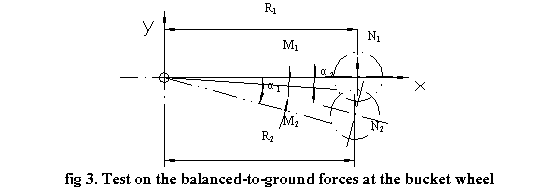Testing condition: within the range of the luffing angles for test, the position of the gravity center of the luffing system must be at the front of the axis of the two pivots on the slewing platform all the time, preventing from overturning back; the cylinder must be off the luffing system; the balanced-to-ground forces tested at the bucket wheel must be over zero. After measuring, the unbalanced torque: M1=N1R1 （1）β=α1, if α1=0  ?nbsp; ( horizontal) M2=N2R2 （2）β=α2 Explanation: β-----------------the luffing angles α1, α2, ? M1, M2----- the unbalanced torques corresponding toα1, α2, kN.m; N1, N2------------ the unbalanced-to-ground forces at the bucket wheel corresponding toα1, α2, kN ; R1, R2------------ the distances in horizontal from N1, N2 to the axis of the two pivots, m; 1.2 Measuring the unbalanced torque by means of testing the pressure of the hydraulic cylinder.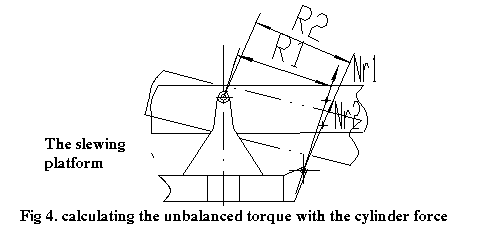Applying pressure gauge with high precision or digital pressure gauge to test pressure (Pa1, Pb1, Pa2, Pb2) on the piston side and piston rod side of cylinder respectively. Calculating the force on the cylinder: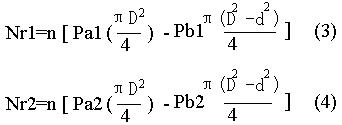Explanation: D-----------------the diameter of piston, m; d-----------------the diameter of piston rod, m; Pa1--------------the pressure on the piston side of cylinder at the luffing angle α1,kPa; Pb1--------------the pressure on the piston rod side of cylinder at the luffing angle α1, kPa; Pa2-----the pressure on the piston side of cylinder at the luffing angle α2, kPa; Pb2-----the pressure on the piston rod side of cylinder at the luffing angle α2, kPa; Nr1, Nr2--------the total force on one or two hydraulic cylinders at the hoisting angle α1 orα2 , kN; when Nr1 > 0 or Nr2 > 0 , the hydraulic cylinder is pushed; when Nr1 < 0 or Nr2 < 0 , the hydraulic cylinder is pulled; n----------------the number of the hydraulic cylinder  Calculating the unbalanced torque: M1=Nr1.R1 (5) β=α1, if:α1=0?horizontal) M2=Nr2.R2 (6) β=α2, Explanation: M1, M2 are the unbalanced torques at the luffing angleα1 orα2 respectively, kN.m; Nr1, Nr2 are the force on one or two hydraulic cylinders at the luffing angleα1 orα2 respectively, kN; R1, R2 are the distances from the axis of the two pivots of the luffing system to the axis of the piston rod at the luffing angleα1 orα2 respectively, m; 2. Calculation on the position of the gravity center of the luffing system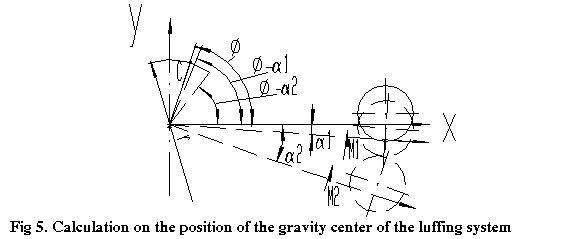After measuring the unbalanced torque, according to two luffing angles α1 andα2 as well as corresponding M1, M2, the position of gravity center is calculated. This gravity center of luffing system is the relevant coordinate position corresponding to the axis of the two pivots on the slewing platform at the hoisting angle 0? Fig 5. is sketch for calculating the gravity center. The coordinate origin ‘o?is the axis of the two pivots supporting the luffing system, angle α1 andα2 are the luffing angles of two positions at the time of luffing down respectively, presuming C the gravity center of luffing system, rotating radium around point ‘o?r, the angle from the line connecting ‘c?and ‘o?to X axis φ. After changing the luffing angle, the angles from the line connecting gravity center ‘c? and the coordinate origin ‘o?to X axis are φ-α1 andφ-α2 respectively. From the static balance relationship: M1=Grgcos(φ-α1) (7) M2=Grgcos(φ-α2) (8) Explanation: G------the total mass of the luffing system, t; r-------the rotating radium when the gravity center luffing, m; g------the gravity acceleration, 9.81m/s2; (7) divided by (8):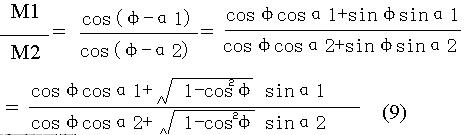If ：E=M1/M2，X=cosφ,a=cosα1 , b= sinα1，c= cosα2，d= sinα2； inserting formula(9):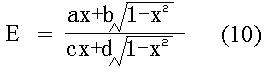clearing up: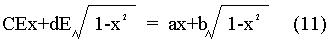moving items: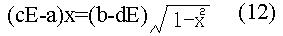squaring the two sides of the equation: ((cE-a)2x2=(b-dE)2(1-x2) (13) moving items: [(cE-a)2+ (b-dE)2 ]x2 = (b-dE)2       (14)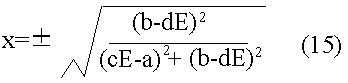inserting the original each variable into formula(15):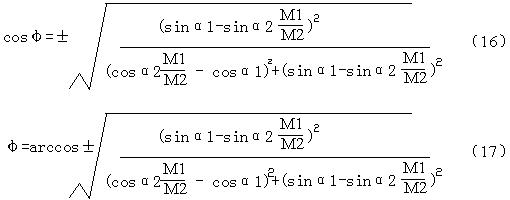when gravity center luffing, the rotating radium around point ‘o? is: r=M1/(Ggcos(φ-α1)) (18) the coordinate of gravity center of the hoisting system (horizontal) is: xc=rcos(φ) (19) yc=rsin(φ) (20) 3. The changing law on the gravity center of the luffing system The position of the gravity center of the luffing system changes in accordance with the luffing angles, the changing law: xc=rcos(φ+α) (21) yc=rsin(φ+α) (22) explanation: α-------------- the luffing angles, at luffing up, α＞0?/p> at luffing down, α＜0?/p> at level, α=0?/p> 4.The significance of calculating the position as well as the changing law of gravity center of the luffing system: a. Analyzing the changing law of force on the cylinder within the whole range of luffing angles furthermore. b. Analyzing the stability of the whole machine as well as the state of traveling wheel pressure furthermore. c. Offering the basis of load changing at different luffing angles for people maintaining the equipment with enough knowledge on it, avoiding fault in work. By liyimin 18 July ,2000